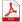# #13

#### The Empirical Probability Distribution Generator (GENPDF)

This component derives the probability distribution functions (PDFs) from the historical record (HURDAT2) that are subsequently used by the STORMGEN track generator. The PDFs are conditional probabilities, as they depend on location, time of season, and other parameters. The PDFs are empirical in that they are obtained by discrete binning. The following PDFs are derived:

• Initial storm speed
• Initial storm direction
• Initial storm intensity (pressure)
• Change in storm speed
• Change in storm angle
• Change in storm intensity (relative intensity)

The bin size and location of these PDFs are defined in a header file “genpdf.h” which is used by both GENPDF and STORMGEN. The bins may be linearly or nonlinearly spaced. A mapping function is available which allows nonlinear mapping so that higher resolution (of a particular parameter) may be obtained. The PDFs for changes in storm motion and intensity are for a 24 hour interval.

Using random initial conditions, storm genesis is defined to occur when a storm first enters or appears within the threat area and has a minimum wind speed of 64 kt. The threat area is described in Section 2.1.2.1. Using specified initial conditions, storm genesis for historical landfalling storms are taken from the track position 36 hours prior to landfall. For non-landfalling storms, the initial condition is taken from the position when the storm first enters the threat area as a hurricane.

The HURDAT2 database contains a variety of storm report types:

• “E” – extra tropical
• “L” – low
• “D” – depression
• “S” – subtropical
• “W” – wave
• tropical – pressure reports
• tropical – wind reports

All non-tropical storm reports (“E”,”L”,”D”,”W”,”S”) are excluded in the intensity PDFs. Pressure reports are used whenever available. If a pressure report is not available, then an attempt is made to interpolate from reports that are within a 24 hour period including the target report. Otherwise, pressure is obtained using an empirical wind-pressure relation (see Appendix A). Intensity changes are only computed for similar report types – observed pressure or wind-derived pressures. Mixing observed and wind-derived pressures was found to create spurious pressure changes. Pressures over land were excluded.

Due to the sparsity of data in some regions or parameter space, the PDFs may be coarsened (bins widened) so that a sufficient number of observations are available to create a robust PDF. This is done in the RESIZE function in GENPDF.

Pressure changes are converted to relative intensity changes. The relative intensity calculation is described in Appendix B. PDFs for pressure and relative intensity are created, though only one is used in STORMGEN. By default, the relative intensity PDF is used by STORMGEN.

Input Data

GENPDF requires the following input files:

• The HURDAT2 database
• Land Mask file – the land mask is based on USGS land use data.
• Outflow temperature for the relative intensity calculation (see Appendix B)
• Sea surface temperatures for the relative intensity calculation (see Appendix B)

Output Data

• Initial storm location, motion, and intensity of all selected storms
• Initial storm location, motion, and intensity PDFs
• Storm motion and intensity change PDFs
• Diagnostic output file

#### Appendix A – Wind-Pressure Relationship

An empirical wind-pressure relation is used to convert HURDAT2 wind reports to pressure. The relation is dependent on region.

The relation is
If longitude is > 81.5W and latitude > 20N,$P = 1013 - ( W / 10.627)^{1.7730}$
Else if latitude < 25 N,$P = 1013 - ( W / 12.016)^{1.8737}$
Else if latitude < 35N,$P = 1013 - ( W / 14.172)^{2.0929}$
Else,$P = 1013 - ( W / 16.086)^{2.3079}$

Where P is central pressure in mb and W is wind speed in kt.

This relationship is described in the report: “The Atlantic Hurricane Database Re-analysis Project – Documentation for 1850-1910 Alterations and Additions to the HURDAT2 Database” by Christopher W. Landsea et al. (contributed as a Chapter for the RPI book (24 January 2002))

#### Appendix B – Relative Intensity Calculation

The relative intensity calculation is based on Darling (1991). The calculation is as follows:

rv= 461rh= 0.80

e= (ts– to) / ts es= 6.112 * exp(17.67 (ts– 273.) / (ts– 29.5))$Pda = 1013 - (rh \cdot es)$$Lv = 2.5 \cdot 10^{6} - 2320 (ts - 273)$$a = ( e \cdot Lv \cdot es) / (( 1 - e ) \cdot rv \cdot ts \cdot Pda )$$b = rh \cdot (1 + es \cdot \log (rh) / (Pda \cdot a ))$

Then solve for x in$x = esp ( -a ( 1 / x - b ))$ and then finally the relative intensity is given by$RI = (1013 - Pmsl + (1 - rh) \cdot es) / (( 1 - x )( 1013 - (rh \cdot es )))$

#### Data Sources

This calculation requires the mean sea level pressure (Pmsl) as input, which in our case is the storm central pressure, the outflow (to) and sea surface temperatures (ts). The outflow temperature is taken to be the monthly mean 100 millibar temperature derived by the Climate Diagnostics Center (CDC) using National Center for Environmental Prediction Center (NCEP) Reanalysis II data. This data is available online at http://www.cdc.noaa.gov/ncep_reanalysis. The sea surface temperature data is monthly mean Reynolds Optimal Interpolation Version 2 (Oiv2) data (Reynolds et al., 2002)Sidebar